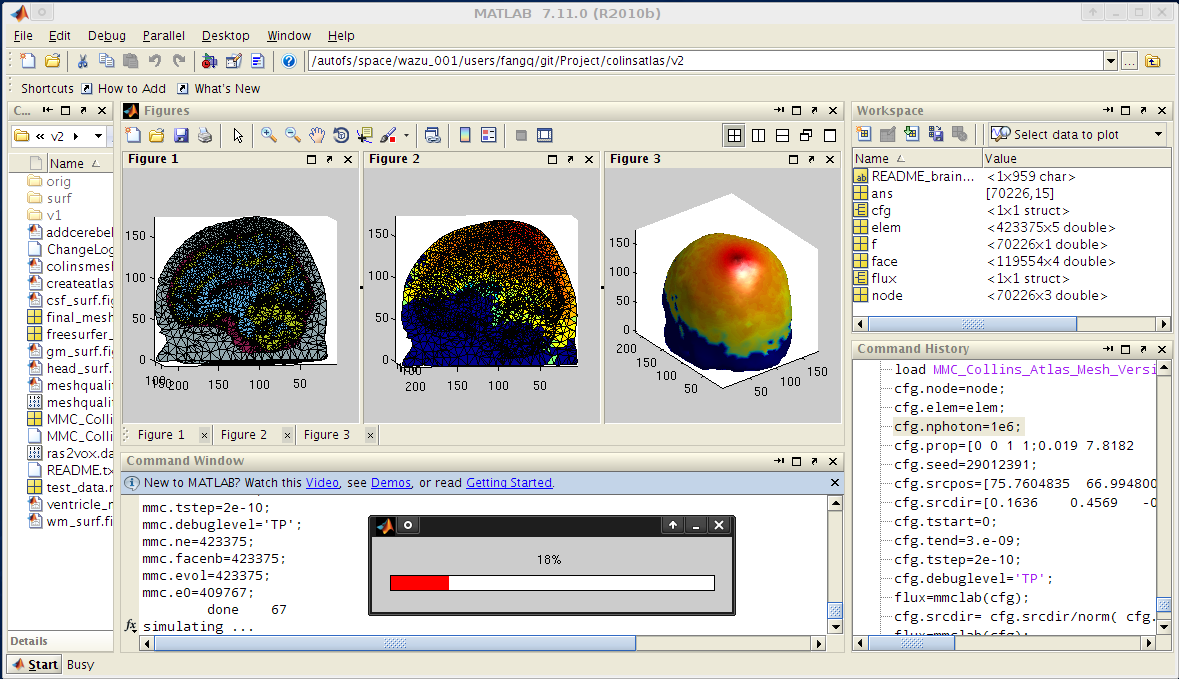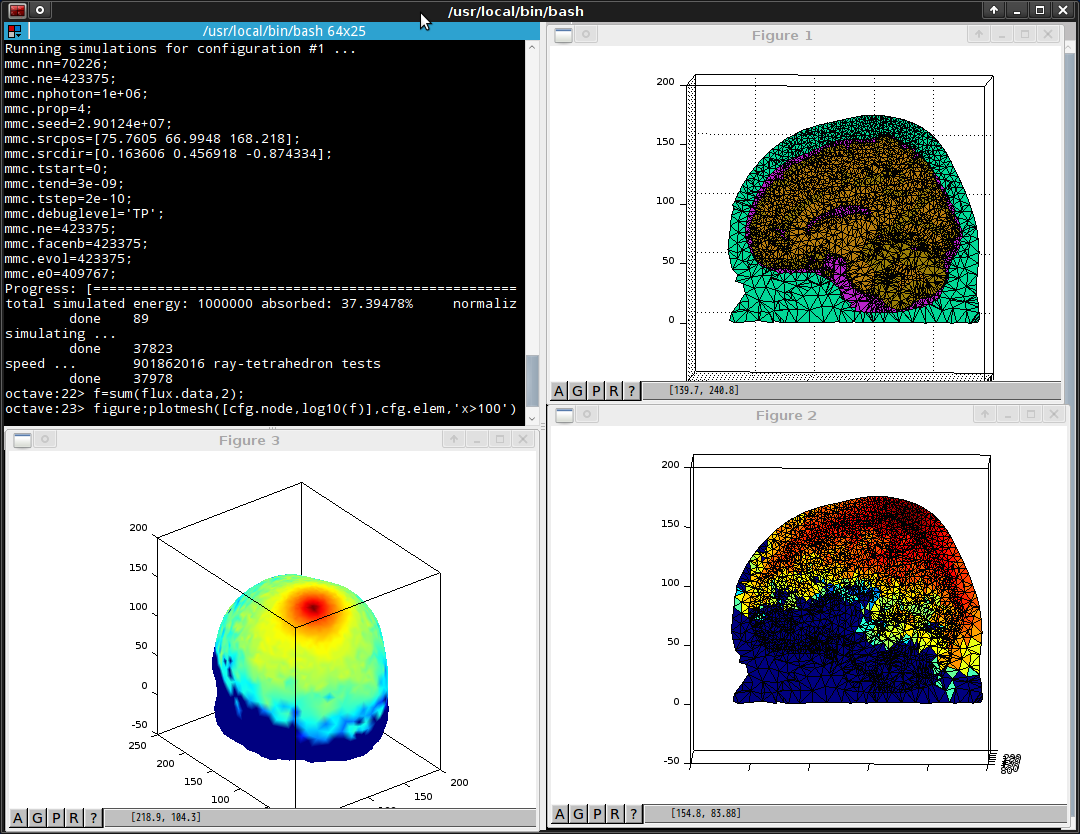# MMCLAB: MMC for MATLAB and GNU Octave

1. Introduction
2. Installation
3. How to use MMCLAB in MATLAB/Octave
4. Examples
5. How to compile MMCLAB
6. Screenshots
7. Reference

## 1. Introduction

MMC is a mesh-based Monte Carlo (MC) photon simulation software. It can utilize a tetrahedral mesh to model a complex anatomical structure, thus, has been shown to be more accurate and computationally efficient than the conventional MC codes.

MMCLAB is the native MEX version of MMC for MATLAB and GNU Octave. By converting the input and output files into convenient in-memory variables, MMCLAB is very intuitive to use and straightforward to be integrated with mesh generation and post-simulation analyses.

Because MMCLAB contains the same computational codes for multi-threading photon simulation as in a MMC binary, running MMCLAB inside MATLAB is expected to give similar speed as running a standalone MMC binary. If your CPU supports, running mmclab with the 'sse' option can be 25% faster than the standard mode.

## 2. Installation

Installation of MMCLAB is straightforward. You first download the MMCLAB package and unzip it to a folder; then you add the folder path into MATLAB's search path list. This can be done with the "addpath" command in a working session; if you want to add this path permanently, use the "pathtool" command, or edit your startup.m (~/.octaverc for Octave).

After installation, please type "help mmclab" in MATLAB/Octave to print the help information.

## 3. How to use MMCLAB in MATLAB/Octave

To learn the basic usage of MMCLAB, you can type

```  help mmclab
```

and enter in MATLAB/Octave to see the help information regarding how to use this function. The help information is listed below. You can find the input/output formats and examples. The input cfg structure has very similar field names as the verbose command line options in MMC.

```%#############################################################################%
%         MMCLAB - Mesh-based Monte Carlo (MMC) for MATLAB/GNU Octave         %
%          Copyright (c) 2010-2015 Qianqian Fang <q.fang at neu.edu>          %
%                            http://mcx.space/mmc/                            %
%                                                                             %
%         Computational Imaging Laboratory (CIL) [http://fanglab.org]         %
%            Department of Bioengineering, Northeastern University            %
%                                                                             %
%               Research funded by NIH/NIGMS grant R01-GM114365               %
%#############################################################################%
%\$Rev::08da9f\$ Last \$Date::2015-04-15 13:39:25 -04\$ by \$Author::Qianqian Fang\$%
%#############################################################################%

Format:
[flux,detphoton,ncfg,seeds]=mmclab(cfg,type);

Input:
cfg: a struct, or struct array. Each element in cfg defines
a set of parameters for a simulation.

It may contain the following fields:

*cfg.nphoton:     the total number of photons to be simulated (integer)
*cfg.prop:        an N by 4 array, each row specifies [mua, mus, g, n] in order.
the first row corresponds to medium type 0 which is
typically [0 0 1 1]. The second row is type 1, and so on.
*cfg.node:        node array for the input tetrahedral mesh, 3 columns: (x,y,z)
*cfg.elem:        element array for the input tetrahedral mesh, 4 columns
*cfg.elemprop:    element property index for input tetrahedral mesh
*cfg.tstart:      starting time of the simulation (in seconds)
*cfg.tstep:       time-gate width of the simulation (in seconds)
*cfg.tend:        ending time of the simulation (in second)
*cfg.srcpos:      a 1 by 3 vector, the position of the source in grid unit
*cfg.srcdir:      a 1 by 3 vector, specifying the incident vector
-cfg.facenb:      element face neighbohood list (calculated by faceneighbors())
-cfg.evol:        element volume (calculated by elemvolume() with iso2mesh)
-cfg.e0:          the element ID enclosing the source, if not defined,
it will be calculated by tsearchn(node,elem,srcpos);
if cfg.e0 is set as one of the following characters,
mmclab will do an initial ray-tracing and move
srcpos to the first intersection to the surface:
'>': search along the forward (srcdir) direction
'<': search along the backward direction
'-': search both directions
cfg.seed:        seed for the random number generator (integer) 
if set as a uint8 array, the binary data in each column is used
to seed a photon (i.e. the "replay" mode)
cfg.detpos:      an N by 4 array, each row specifying a detector: [x,y,z,radius]
cfg.isreflect:   -consider refractive index mismatch, 0-matched index
cfg.isnormalized:-normalize the output flux to unitary source, 0-no reflection
cfg.isspecular:  -calculate specular reflection if source is outside
cfg.ismomentum:  -save momentum transfer for each detected photon
cfg.issaveexit:  -save the position (x,y,z) and (vx,vy,vz) for a detected photon
cfg.issaveseed:  -save the RNG seed for a detected photon so one can replay
cfg.basisorder:  -linear basis, 0-piece-wise constant basis
cfg.outputformat:['ascii'] or 'bin' (in 'double')
cfg.outputtype:  [X] - output flux, F - fluence, E - energy deposit
J - Jacobian (replay), T - approximated Jacobian (replay mode)
cfg.method:      ray-tracing method, [P]:Plucker, H:Havel (SSE4),
cfg.debuglevel:  debug flag string, a subset of [MCBWDIOXATRPE], no space
cfg.nout:        [1.0] refractive index for medium type 0 (background)
cfg.minenergy:   terminate photon when weight less than this level (float) [0.0]
cfg.roulettesize: size of Russian roulette
cfg.unitinmm:    defines the unit in the input mesh [1.0]
cfg.srctype:     source type, the parameters of the src are specified by cfg.srcparam{1,2}
'pencil' - default, pencil beam, no param needed
'isotropic' - isotropic source, no param needed
'cone' - uniform cone beam, srcparam1(1) is the half-angle in radian
'gaussian' - a collimated gaussian beam, srcparam1(1) specifies the waist radius (in voxels)
'planar' - a 3D quadrilateral uniform planar source, with three corners specified
by srcpos, srcpos+srcparam1(1:3) and srcpos+srcparam2(1:3)
'pattern' - a 3D quadrilateral pattern illumination, same as above, except
srcparam1(4) and srcparam2(4) specify the pattern array x/y dimensions,
and srcpattern is a pattern array, valued between [0-1].
'fourier' - spatial frequency domain source, similar to 'planar', except
the integer parts of srcparam1(4) and srcparam2(4) represent
the x/y frequencies; the fraction part of srcparam1(4) multiplies
2*pi represents the phase shift (phi0); 1.0 minus the fraction part of
srcparam2(4) is the modulation depth (M). Put in equations:
S=0.5*[1+M*cos(2*pi*(fx*x+fy*y)+phi0)], (0<=x,y,M<=1)
'arcsine' - similar to isotropic, except the zenith angle is uniform
distribution, rather than a sine distribution.
'disk' - a uniform disk source pointing along srcdir; the radius is
set by srcparam1(1) (in grid unit)
'fourierx' - a general Fourier source, the parameters are
srcparam1: [v1x,v1y,v1z,|v2|], srcparam2: [kx,ky,phi0,M]
normalized vectors satisfy: srcdir cross v1=v2
the phase shift is phi0*2*pi
'fourierx2d' - a general 2D Fourier basis, parameters
srcparam1: [v1x,v1y,v1z,|v2|], srcparam2: [kx,ky,phix,phiy]
the phase shift is phi{x,y}*2*pi
'zgaussian' - an angular gaussian beam, srcparam1(0) specifies the variance in the zenith angle
cfg.{srcparam1,srcparam2}: 1x4 vectors, see cfg.srctype for details
cfg.srcpattern: see cfg.srctype for details
cfg.voidtime:   for wide-field sources, -start timer at launch, 0-when entering
the first non-zero voxel

fields marked with * are required; options in [] are the default values
fields marked with - are calculated if not given (can be faster if precomputed)

type: omit or 'omp' for multi-threading version; 'sse' for the SSE4 MMC,
if type='prep' with a single output, mmclab returns ncfg only.

Output:
flux: a struct array, with a length equals to that of cfg.
For each element of flux, flux(i).data is a 1D vector with
dimensions [size(cfg.node,1) total-time-gates] if cfg.basisorder=1,
or [size(cfg.elem,1) total-time-gates] if cfg.basisorder=0.
The content of the array is the normalized flux (or others
depending on cfg.outputtype) at each mesh node and time-gate.
detphoton: (optional) a struct array, with a length equals to that of cfg.
For each element of detphoton, detphoton(i).data is a 2D array with
dimensions [size(cfg.prop,1)+1 saved-photon-num]. The first row
is the ID(>0) of the detector that captures the photon; the second row
is the number of scattering events of the exitting photon; the rest rows
are the partial path lengths (in grid unit) traveling in medium 1 up
to the last. If you set cfg.unitinmm, you need to multiply the path-lengths
to convert them to mm unit.
ncfg: (optional), if given, mmclab returns the preprocessed cfg structure,
including the calculated subfields (marked by "-"). This can be
used as the input to avoid repetitive preprocessing.
seeds: (optional), if give, mmclab returns the seeds, in the form of
a byte array (uint8) for each detected photon. The column number
of seed equals that of detphoton.

Example:
cfg.nphoton=1e5;
[cfg.node face cfg.elem]=meshabox([0 0 0],[60 60 30],6);
cfg.elemprop=ones(size(cfg.elem,1),1);
cfg.srcpos=[30 30 0];
cfg.srcdir=[0 0 1];
cfg.prop=[0 0 1 1;0.005 1 0 1.37];
cfg.tstart=0;
cfg.tend=5e-9;
cfg.tstep=5e-10;
cfg.debuglevel='TP';
% populate the missing fields to save computation
ncfg=mmclab(cfg,'prep');

cfgs(1)=ncfg;
cfgs(2)=ncfg;
cfgs(1).isreflect=0;
cfgs(2).isreflect=1;
cfgs(2).detpos=[30 20 0 1;30 40 0 1;20 30 1 1;40 30 0 1];
% calculate the flux and partial path lengths for the two configurations
[fluxs,detps]=mmclab(cfgs);

This function is part of Mesh-based Monte Carlo (MMC) URL: http://mcx.sf.net/mmc/

```

## 4. Examples

We provided several examples to demonstrate the basic usage of MMCLAB; some of the examples also perform validations of MMC algorithm in both homogeneous and heterogeneous domains. These examples are explained below:

#### demo_mmclab_basic.m

In this example, we show the most basic usage of MMCLAB. This includes how to define the input configuration structure, launch MMC simulations and plot the output data.

#### demo_example_validation.m

In this example, we validate MMCLAB with a homogeneous medium in a cubic domain. This is the same as in mmc/examples/validation; the details of the simulation is described in Fig.2 of [Fang2010].

#### demo_example_meshtest.m

In this example, we validate the MMCLAB solver with a heterogeneous domain and the analytical solution from the diffusion model. The domain is consisted of a 6x6x6 cm box with a 2cm diameter sphere embedded at the center.

This test is identical to the simulations under mmc/examples/meshtest, which are described by Fig. 3 in [Fang2010].

#### demo_example_onecube.m

In this example, we demonstrate how to use the debug flags to print photon trajectories. The domain is a simple 10x10x10mm cube.

This test is similar to the simulations under mmc/examples/onecube.

#### demo_example_replay.m

In this example, we run MMC using a homogeneous cubic domain, same as in demo_example_validation.m. We save the seeds of the detected photons, and then rerun these photons again (i.e. the "replay" part). This feature allows one to identify features that are only related to a source/detector pair.

#### demo_compare_mmc_mcx.m

In this example, we compare MMC and MCX using both simple and widefield sources.

#### demo_sfdi_2layer.m

In this example, we simulate an spatial-frequency domain imaging source using a 2-layer brain model.

## 5. How to compile MMCLAB

To compile MMCLAB from source code, you need to make sure your computer have the following requirements:

• your computer should have MATLAB C compiler (mex) and/or octave3.x-headers installed
• to compile the SSE versions, your computer should support SSE4 instructions
• the commands "mex" and "mkoctfile" should be in the search path (\$PATH)
• you should have both gcc and g++ with version 4.4 or newer
• for windows, you should install MinGW32 with gcc/g++ via mingw-get-inst
• for windows, you need to create a mexopts.bat file as C:\Users\<username>\AppData\Roaming\MathWorks\MATLAB\RXXXX\mexopts.bat with content modified from https://www.dynare.org/trac/browser/windows/mexopts-win64.bat?rev=fd693204f929a11378f78de6c5e750bc094c71d5

To compile MMCLAB for MATLAB, you need to cd mmc/src directory, and type

``` make mex
```
or
``` make mexsse
```

from a shell window. You need to make sure your MATLAB is installed and the command mex is included in your PATH environment variable. Similarly, to compile MMCLAB for Octave, you type

``` make oct
```
or
``` make octsse
```

The command mkoctfile must be accessible from your command line and it is provided in a package named "octave3.x-headers" in Ubuntu (3.x can be 3.2 or 3.4 etc).

Compiling MMCLAB on Windows and Mac OS, you should use the following command:

``` make ... -f makefile_sfmt ...
```

If you have multiple gcc/g++ installed on your system, and the default gcc and g++ is older than 4.4 (use gcc -v to print version), you can use the following command to specify the correct gcc command:

``` make ... CC=/path/to/gcc/4.4 CXX=/path/to/g++/4.4
```

## 6. Screenshots

Screenshot for using MMCLAB in MATLAB:

``````

Screenshot for using MMCLAB in GNU Octave:

``````

## 7. Reference

``` [Fang2010] Fang Q, "Mesh-based Monte Carlo method using fast ray-tracing
in Plucker coordinates," Biomed. Opt. Express 1(1), 165-175 (2010)
```